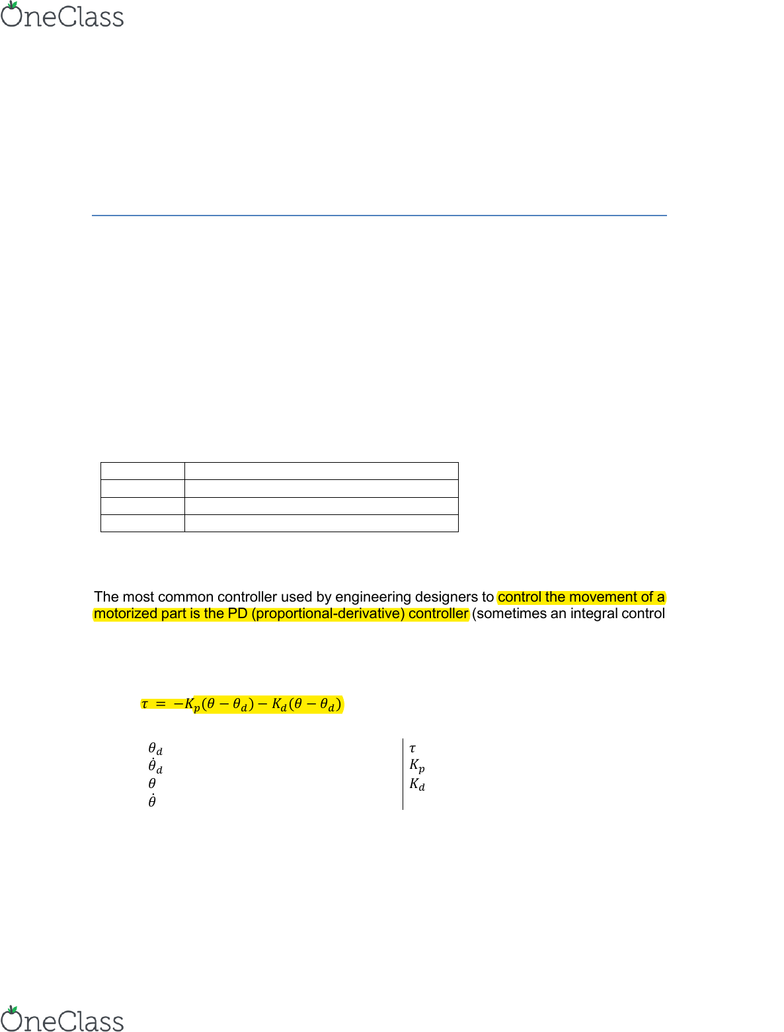# ENGRMAE 106 Lecture Notes - Lecture 1: Angular Velocity, Damping Ratio, Step Response

24 views7 pages
Page:
of 7MAE106 Laboratory Exercises
Lab # 5
PD Control of DC motor position
University of California, Irvine
Department of Mechanical and Aerospace Engineering
Goals
Understand how to implement and tune a PD controller to control the position of a DC
motor.
Explore the frequency response of the PD controller by testing how it responds to
sinusoidal inputs of different frequencies.
Parts & equipment
Qty
Part/Equipment
1
Seeeduino board
1
Motor driver
1
DC motor with encoder
Introduction to PD Control
The most common controller used by engineering designers to control the movement of a
motorized part is the PD (proportional-derivative) controller (sometimes an integral control
term is added to create a PID controller, but we will not explore I control in this lab). In this
lab you will implement a PD position controller. Such controllers are also used in robot
arms, radars, numerically controlled milling machines, manufacturing systems, and control
surfaces on aerospace vehicles. The PD control law is:
where:
desired motor angular position
desired motor torque
desired motor angular velocity
proportional gain
actual motor angular position
derivative gain
actual motor angular velocity
Note that the controller has two terms one proportional to the position error (the Ppart),
and one proportional to the derivative of position (i.e. velocity, the “D” part). Thus, it is
called a “PD” controller. The implementation of this controller for a DC motor with inertia,
J, is shown in Figure 2.
find more resources at oneclass.com
find more resources at oneclass.com
Figure 1. Block diagram that you will implement to make the PD controller for the motor. J
is the inertia of the motor shaft.
To understand how the actual system behaves we need to first understand its dynamics.
First, let's look at the dynamical equation that describes how θ evolves with time when the
controller is attached to the motor.
Dynamics of the motor and shaft:
  
Dynamics of the controller system:
          
Re-writing to make input-output clear:

 
   
This differential equation has similar dynamics to a mass-spring-damper system with
Force as the input and Position as the output. That is, it follows the same equations of
motion. This allows us to use our intuition about mass-spring-damper systems when
designing and tuning a PD controller.
Recall that the differential equation of motion for a mass-spring-damper system is given
by:

and thus using the analogy to the PD controller we have that:
mass-spring-damper system
PD controller
mass
J
motor inertia
damper
derivative control term
spring
proportional control term
input force
desired motor torque
find more resources at oneclass.com
find more resources at oneclass.com
In a mechanical system, if you wanted the system to respond more quickly, you would
increase the natural frequency n) by picking a stiffer spring (higher K). Which variable
would you change in your differential equation for the PD system to make your system
respond more quickly (i.e. increase its natural frequency)?
Note: there is a limit to how big you can make this variable because of the time delays in
this sampled data system.
By adding the derivative gain (Kd) to control the position of the motor we must now take
into account the concept of damping when designing and implementing the controller.
With damping in the controller we can have four types of behaviors:
Undamped (i.e. zero damping): The system oscillates at its natural frequency.
These oscillations are a function of the controller's gain, Kp.